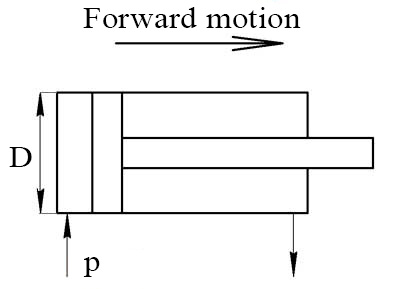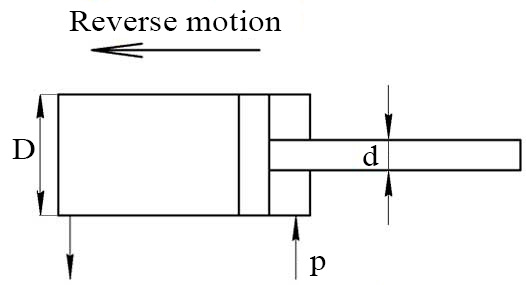Hydraulic cylinder force calculator

The cylinder force F is dependent on the operating pressure p and the effective area A.

F = pÃ—A

The force produced on the opposite of rod side

The calculated equation:

F = p×πD2/4Pressure p: Units Pa Pascal kPa KiloPascal MPa MegaPascal Bar Bar psi Pound-force per square inch at Technical atmosphere Piston diameter D: Units mm Millimetre cm Centimeter dm Decimeter m Meter km Kilometer in Inch

The force produced on the rod side

Equation for calculating the force of the double action hydraulic cylinder in the reverse motion:

F = p×π(D2-d2)/4Pressure p: Units Pa Pascal kPa KiloPascal MPa MegaPascal Bar Bar psi Pound-force per square inch at Technical atmosphere Piston diameter D: Rod diameter d: Units mm Millimetre cm Centimeter dm Decimeter m Meter km Kilometer in Inch

Languages
Spanish (ES) Deutsch (DE)
Featured articles